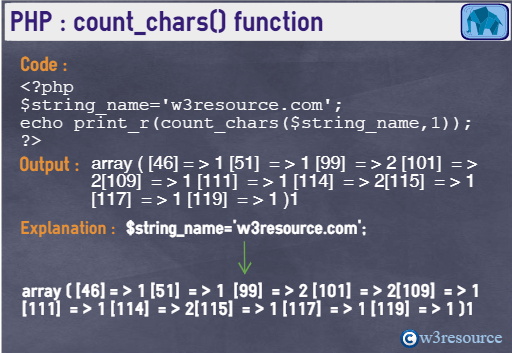# PHP: count_chars() function

## Description

The count_chars() function is used to count the number of times that an ASCII character occurs within a string

Version:

(PHP 4 , PHP 5)

Syntax:

`count_chars(string1, mode)`

Parameters:

Name Description Required /
Optional
Type
String1 The specified string. Required Float
mode The return mode. 0 - an array with the byte value as key and the frequency of every byte as value. 1 - same as 0 but only byte values with a frequency greater than zero are listed. 2 - same as 0 but only byte values with a frequency equal to zero are listed. 3 - a string containing all used byte-values is returned. 4 - a string containing all not used byte-values is returned. Optional Integer

Return values

Depending on mode.

Value Type: Mixed*.

*Mixed: Mixed indicates that a parameter may accept multiple (but not necessarily all) types.

Pictorial PresentationExample:

``````<?php
\$string_name='w3resource.com';
echo print_r(count_chars(\$string_name,0));
?>
``````

Output :

`Array  (       => 0       => 0       => 0       => 0       => 0       => 0       => 0       => 0       => 0       => 0       => 0       => 0       => 0       => 0       => 0       => 0       => 0       => 0       => 0       => 0       => 0       => 0       => 0       => 0       => 0       => 0       => 0       => 0       => 0       => 0       => 0       => 0       => 0       => 0       => 0       => 0       => 0       => 0       => 0       => 0       => 0       => 0       => 0       => 0       => 0       => 0       => 1       => 0       => 0       => 0       => 0       => 1       => 0       => 0       => 0       => 0       => 0       => 0       => 0       => 0       => 0       => 0       => 0       => 0       => 0       => 0       => 0       => 0       => 0       => 0       => 0       => 0       => 0       => 0       => 0       => 0       => 0       => 0       => 0       => 0       => 0       => 0       => 0       => 0       => 0       => 0       => 0       => 0       => 0       => 0       => 0       => 0       => 0       => 0       => 0       => 0       => 0       => 0       => 0       => 2       => 0       => 2       => 0       => 0       => 0       => 0       => 0       => 0       => 0       => 1       => 0       => 2       => 0       => 0       => 2       => 1       => 0       => 1       => 0       => 1       => 0       => 0       => 0       => 0       => 0       => 0       => 0       => 0       => 0       => 0       => 0       => 0       => 0       => 0       => 0       => 0       => 0       => 0       => 0       => 0       => 0       => 0       => 0       => 0       => 0       => 0       => 0       => 0       => 0       => 0       => 0       => 0       => 0       => 0       => 0       => 0       => 0       => 0       => 0       => 0       => 0       => 0       => 0       => 0       => 0       => 0       => 0       => 0       => 0       => 0       => 0       => 0       => 0       => 0       => 0       => 0       => 0       => 0       => 0       => 0       => 0       => 0       => 0       => 0       => 0       => 0       => 0       => 0       => 0       => 0       => 0       => 0       => 0       => 0       => 0       => 0       => 0       => 0       => 0       => 0       => 0       => 0       => 0       => 0       => 0       => 0       => 0       => 0       => 0       => 0       => 0       => 0       => 0       => 0       => 0       => 0       => 0       => 0       => 0       => 0       => 0       => 0       => 0       => 0       => 0       => 0       => 0       => 0       => 0       => 0       => 0       => 0       => 0       => 0       => 0       => 0       => 0       => 0       => 0       => 0       => 0       => 0       => 0       => 0       => 0       => 0       => 0       => 0       => 0       => 0       => 0       => 0       => 0       => 0       => 0       => 0  )  1`

View the example in the browser

PHP Function Reference

Previous: convert_uuencode
Next: crc32

﻿

## PHP: Tips of the Day

In PHP, there are two versions of logical AND and OR operators.

Operator True if
\$a and \$b Both \$a and \$b are true
\$a && \$b Both \$a and \$b are true
\$a or \$b Either \$a or \$b is true
\$a || \$b Either \$a or \$b is true

Note that the && and || opererators have higher precedence than and and or. See table below:

Evaluation Result of \$e Evaluated as
\$e = false || true True \$e = (false || true)
\$e = false or true False (\$e = false) or true

Because of this it's safer to use && and || instead of and and or.